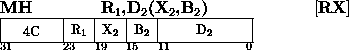Next: OR Up: Insert Character Previous: Multiply

### Multiply HalfwordThe product of the multiplier (second operand) and multiplicand (first operand) replaces the multiplicand. The second operand is two bytes in length and is considered to be a 16-bit signed integer.

Both multiplicand and product are 32-bit signed integers and may be located in any general register. The 16-bit multiplier is expanded to 32 bits before multiplication by propagating the six-bit value through the 16 high-order bit positions. The multiplicand is replaced by the low-order part of the product. The bits to the left of the 32 low-order bits are not tested for significance; no overflow indication is given.

The sign of the product is determined by the rules of algebra from the multiplier and multiplicand sign, except that a zero result is always positive.

Resulting Condition Code:
The code remains unchanged.

Program Exceptions:
Access (fetch, operand 2)

Programming Note
The significant part of the product usually occupies 46 bits or fewer, the exception being 47 bits when both operands are maximum negative. Since the low-order 32 bits of the product are stored unchanged, ignoring all bits to the left, the sign bit of the result may differ from the true sign of the product in the case of overflow.Next: OR Up: Insert Character Previous: Multiply

A.Daviel,TRIUMF